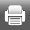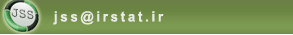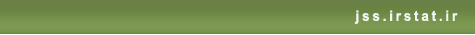[Home ] [Archive]   [ فارسی ]Back to the articles list Back to browse issues page
Randomized Item Count Model
Abstract:   (2349 Views)

In many sample surveys, the variables of interest, such as student cheating in a university are sensitive in nature. In such situations, the interviewees respond to direct questions untruthful, or refuse to answer. The various indirect methods such as randomized response technique and item count technique are introduced to collect sensitive information. In this paper a new item count is proposed, then its randomized version called randomized item count model is introduced. Using this model an unbiased estimator for the sensitive proportion of the population is obtained. The variance of the estimator and an estimate for its variance are obtained. A criterion for comparing efficiency and privacy is introduced simultaneously. Using simulation, the proposed model is evaluated and its efficiency and privacy are compared with the Simons’ technique. Based on this criterion, it is shown that the proposed method is better than the Simons method. The proportion of student cheating in the Shahid Chamran University of Ahvaz is estimated using the proposed model.

Keywords: Randomized Response, Item Count Technique, Sensitive Variable, Proportion of Student Cheating, Randomized Item Count.
Type of Study: Research | Subject: Sampling